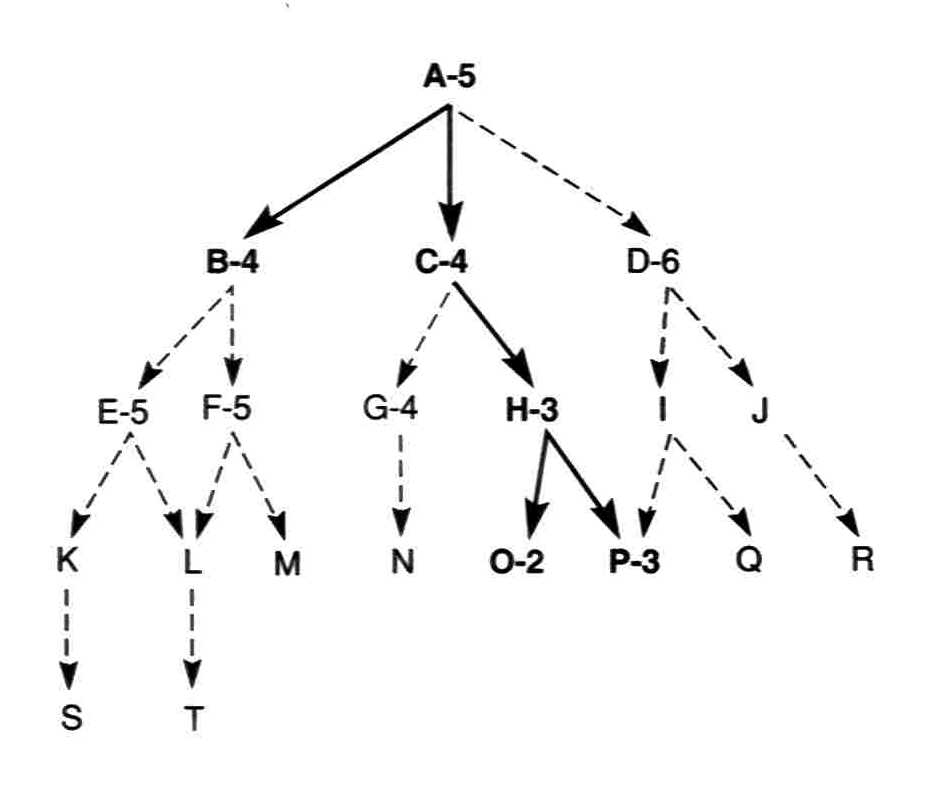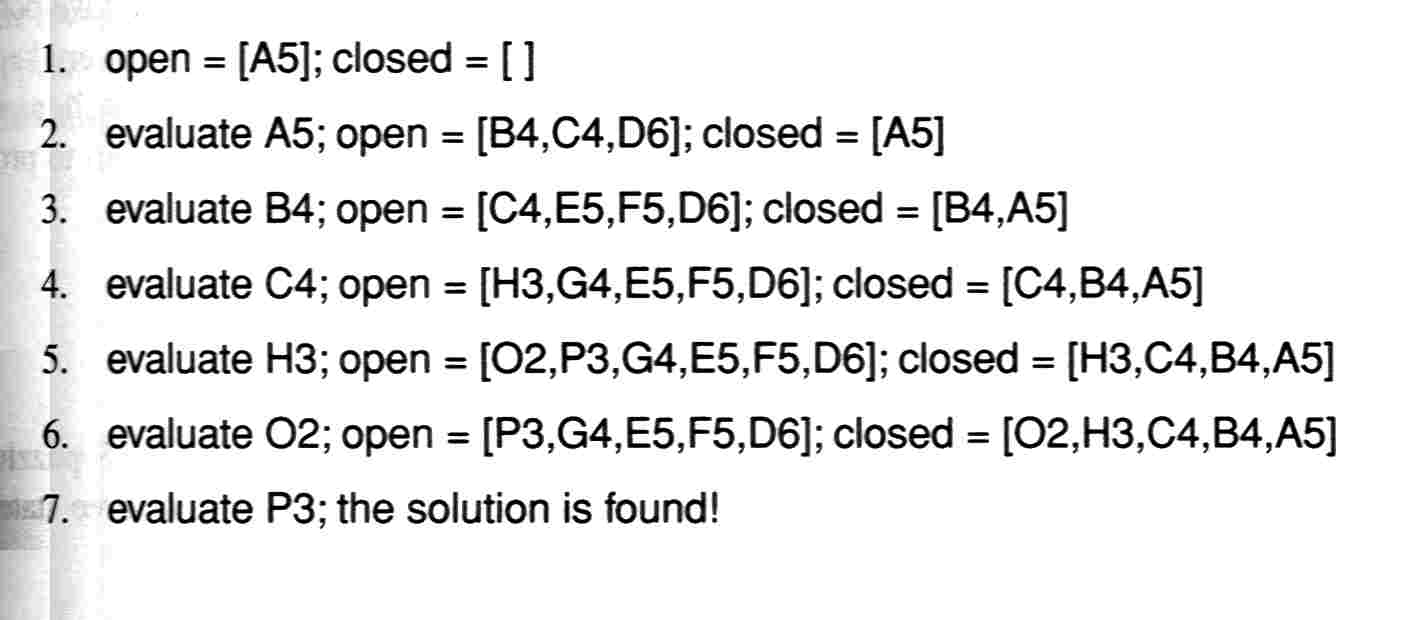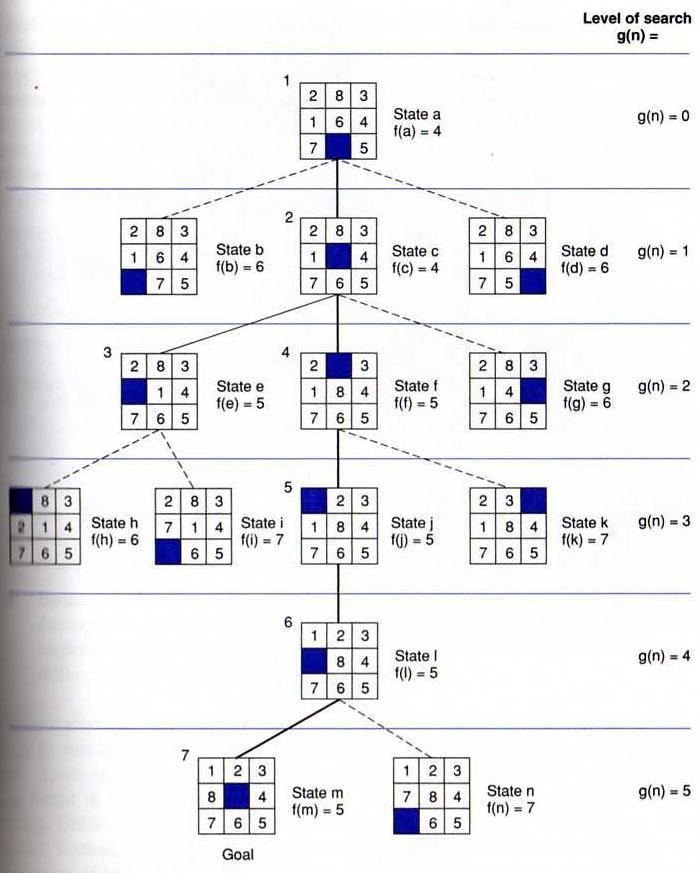3203. Introduction to Artificial Intelligence

Heuristic Search

### 1. Best-first search

Both depth-first search and breadth-first search belong to "uninformed search", where there is no information about the intermediate nodes, so the search is guided only by the structure of the graph.

The other extreme is "completely-informed search", where at each node the best option is known. Actually in such a case no "search" is really needed, since the path from the initial state to the final state is known.

Both above cases can be handled well in computer science. AI is interested in the situation in the middle: the intermediate nodes do have information on how promising each option is, though the information is not always accurate and certain.

A "heuristic function" is a function used to rank the alternatives in each step according to available information. Such a function is usually based on experience and intuition about the given problem.

Heuristic search is similar to depth-first search and breadth-first search, except that its "open-node list" is a priority queue, in which the nodes are sorted by a heuristic function. Consequently in every step the algorithm explores the current-best direction, therefore it is also called "best-first search". The actual efficiency of such an algorithm is determined by the quality of the heuristic function.

Beside looking for a path to a goal state, search can also be used to solve optimization problems, where each state has an evaluation value attached, and the problem is to find the state with the highest value. In this situation, local (i.e., without memory) best-first search is also called "hill climbing". Such a procedure stops at local maxima.

A well-known example is the General Problem Solver, which uses Means-Ends Analysis to get a heuristic function.

### 2. A* Algorithm

A* algorithm is a typical heuristic search algorithm, in which the heuristic function is an estimated shortest distance from the initial state to the closest goal state, and it equals to the traveled distance plus the predicted distance ahead. That is, f(n) = g(n) + h(n).

1. Create a search graph, G, consisting solely of the start node N1. Put N1 in a list called OPEN.
2. Create a list called CLOSED that is initially empty.
3. If OPEN is empty, exit with failure.
4. Select the first node on OPEN, remove it from OPEN, and put it on CLOSED. Call this node N.
5. If N is a goal node, exit successfully with the solution obtained by tracing a path along the pointers from N to N1 in G. (The pointers define a search tree and are established in step 7.)
6. Expand node N, generating the set, M, of its successors that are not already ancestors of N in G. Install these members of M as successors of N in G.
7. Establish a pointer to N from each of those members of M that were not already in G (i.e., not already on either OPEN or CLOSED). Add these members of M to OPEN. For each member, Mi, of M that was already on OPEN or CLOSED, redirect its pointer to N if the best path to Mi found so far is through N. For each member of M already on CLOSED, redirect the pointers of each of its descendants in G so that they point backward along the best paths found so far to these descendants.
8. Reorder the list OPEN in order of increasing f values. (Ties among minimal f values are resolved in favor of the deepest node in the search tree.)
9. Go to step 3.
An example:### 3. Example: 8-puzzle

In the following example, the graph is searched with h(n) as the number of tiles out of place.Another common heuristic function is the sum of the distances of the tiles from their goal positions.

### 4. Heuristic search in Prolog

Here is an A* Algorithm in Prolog, which can solve 8-puzzle by heuristic search.

Other sample Prolog implementations of best-first search: Program 1, Program 2.